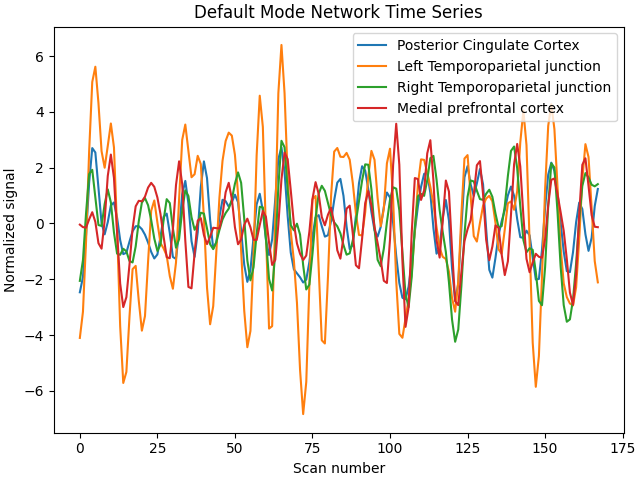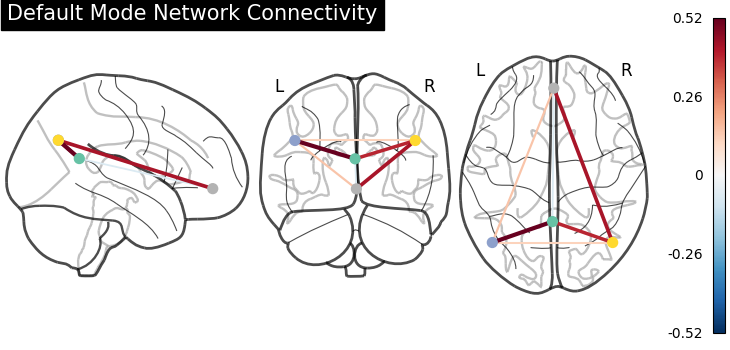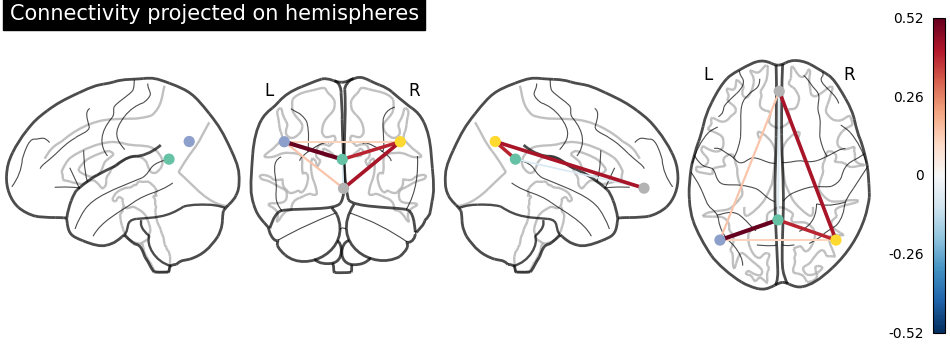# Extract signals on spheres and plot a connectome#

This example shows how to extract signals from spherical regions. We show how to build spheres around user-defined coordinates, as well as centered on coordinates from the Power-264 atlas , and the Dosenbach-160 atlas .

Note

If you are using Nilearn with a version older than `0.9.0`, then you should either upgrade your version or import maskers from the `input_data` module instead of the `maskers` module.

That is, you should manually replace in the following example all occurrences of:

```from nilearn.maskers import NiftiMasker
```

with:

```from nilearn.input_data import NiftiMasker
```

References

 Power, Jonathan D., et al. “Functional network organization of the human brain.” Neuron 72.4 (2011): 665-678.

 Dosenbach N.U., Nardos B., et al. “Prediction of individual brain maturity using fMRI.”, 2010, Science 329, 1358-1361.

We estimate connectomes using two different methods: sparse inverse covariance and partial_correlation, to recover the functional brain networks structure.

We’ll start by extracting signals from Default Mode Network regions and computing a connectome from them.

## Retrieve the brain development fmri dataset#

We are going to use a subject from the development functional connectivity dataset.

```from nilearn import datasets

dataset = datasets.fetch_development_fmri(n_subjects=10)

# print basic information on the dataset
print(f"First subject functional nifti image (4D) is at: {dataset.func}")
```
```First subject functional nifti image (4D) is at: /home/runner/work/nilearn/nilearn/nilearn_data/development_fmri/development_fmri/sub-pixar123_task-pixar_space-MNI152NLin2009cAsym_desc-preproc_bold.nii.gz
```

## Coordinates of Default Mode Network#

```dmn_coords = [(0, -52, 18), (-46, -68, 32), (46, -68, 32), (1, 50, -5)]
labels = [
"Posterior Cingulate Cortex",
"Left Temporoparietal junction",
"Right Temporoparietal junction",
"Medial prefrontal cortex",
]
```

## Extracts signal from sphere around DMN seeds#

We can compute the mean signal within spheres of a fixed radius around a sequence of (x, y, z) coordinates with the object `nilearn.maskers.NiftiSpheresMasker`. The resulting signal is then prepared by the masker object: Detrended, band-pass filtered and standardized to 1 variance.

```from nilearn.maskers import NiftiSpheresMasker

dmn_coords,
detrend=True,
standardize="zscore_sample",
standardize_confounds="zscore_sample",
low_pass=0.1,
high_pass=0.01,
t_r=2,
memory="nilearn_cache",
memory_level=1,
verbose=2,
clean__butterworth__padtype="even",  # kwarg to modify Butterworth filter
)

# Additionally, we pass confound information to ensure our extracted
# signal is cleaned from confounds.

func_filename = dataset.func
confounds_filename = dataset.confounds

func_filename, confounds=[confounds_filename]
)
```
```________________________________________________________________________________
{ 'allow_overlap': False,
'detrend': True,
'dtype': None,
'high_pass': 0.01,
'high_variance_confounds': False,
'low_pass': 0.1,
'seeds': [(0, -52, 18), (-46, -68, 32), (46, -68, 32), (1, 50, -5)],
'smoothing_fwhm': None,
'standardize': 'zscore_sample',
'standardize_confounds': 'zscore_sample',
_______________________________________________filter_and_extract - 1.9s, 0.0min
```

## Display time series#

```import matplotlib.pyplot as plt

for time_serie, label in zip(time_series.T, labels):
plt.plot(time_serie, label=label)

plt.title("Default Mode Network Time Series")
plt.xlabel("Scan number")
plt.ylabel("Normalized signal")
plt.legend()
plt.tight_layout()
```## Compute partial correlation matrix#

Using object `nilearn.connectome.ConnectivityMeasure`: Its default covariance estimator is Ledoit-Wolf, allowing to obtain accurate partial correlations.

```from nilearn.connectome import ConnectivityMeasure

connectivity_measure = ConnectivityMeasure(
kind="partial correlation",
standardize="zscore_sample",
)
partial_correlation_matrix = connectivity_measure.fit_transform([time_series])[
0
]
```

## Display connectome#

We display the graph of connections with :func: nilearn.plotting.plot_connectome.

```from nilearn import plotting

plotting.plot_connectome(
partial_correlation_matrix,
dmn_coords,
title="Default Mode Network Connectivity",
)
``````<nilearn.plotting.displays._projectors.OrthoProjector object at 0x7fd0ecc61f10>
```

Display connectome with hemispheric projections. Notice (0, -52, 18) is included in both hemispheres since x == 0.

```plotting.plot_connectome(
partial_correlation_matrix,
dmn_coords,
title="Connectivity projected on hemispheres",
display_mode="lyrz",
)

plotting.show()
```## 3D visualization in a web browser#

An alternative to `nilearn.plotting.plot_connectome` is to use `nilearn.plotting.view_connectome`, which gives more interactive visualizations in a web browser. See 3D Plots of connectomes for more details.

```view = plotting.view_connectome(partial_correlation_matrix, dmn_coords)

# In a Jupyter notebook, if ``view`` is the output of a cell, it will
# be displayed below the cell
view
```